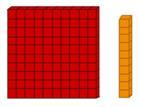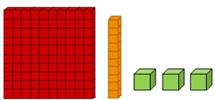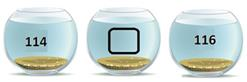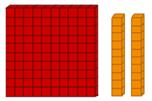Courses

# Test: 3-Digit Numbers- 2

## 10 Questions MCQ Test Mathematics for Class 2 | Test: 3-Digit Numbers- 2

Description
This mock test of Test: 3-Digit Numbers- 2 for Class 2 helps you for every Class 2 entrance exam. This contains 10 Multiple Choice Questions for Class 2 Test: 3-Digit Numbers- 2 (mcq) to study with solutions a complete question bank. The solved questions answers in this Test: 3-Digit Numbers- 2 quiz give you a good mix of easy questions and tough questions. Class 2 students definitely take this Test: 3-Digit Numbers- 2 exercise for a better result in the exam. You can find other Test: 3-Digit Numbers- 2 extra questions, long questions & short questions for Class 2 on EduRev as well by searching above.
QUESTION: 1

### Which of the following numbers is represented by using the given blocks?Solution:

We use 1 hundred block, 1 ten block and 0 ones block to show the number 110.

QUESTION: 2

### Which of the following models will you use to show the number that comes just after 104?

Solution:

105 comes just after 104.
We use one hundred block and five ones block to show number 105.

QUESTION: 3

### Which of the following numbers comes before the number that is shown using the given blocks?Solution:

There is one hundred block, one ten block and three ones block.
So, the model shows 113.
112 comes before 113.
Hence, the answer is one hundred twelve.

QUESTION: 4

Which number comes between 114 and 116?Solution:

One hundred fifteen comes between 114 and 116.

QUESTION: 5

Which of the following is the number name of 118?

Solution:

118 is written in words as one hundred eighteen.

QUESTION: 6

Which of the following numbers can be written as one hundred forty-nine?

Solution:

We write one hundred forty-nine using digits as 149.

QUESTION: 7

Which of the following numbers can be written as one hundred sixty-nine?

Solution:

The number name of 169 is one hundred sixty-nine.

QUESTION: 8

Which of the following is the number name for the number 135?

Solution:

The number name of 135 is one hundred thirty-five.

QUESTION: 9

Which of the following models show the number that comes between 154 and 156?

Solution:
• 155 comes between 154 and 156.
• We use one hundred block, five tens block and 5 ones block to show number 155.
QUESTION: 10

Which of the following numbers is represented using the given blocks?Solution:

We use one hundred block, two tens block and zero ones blocks to show 120.